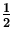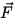Kinetic Energy and the Work Energy Theorem

Idea: Force is a vector, work and energy are scalars. Thus, it is often easier to solve problems using energy considerations instead of using Newton's laws (i.e. it is easier to work with scalars than vectors).

Definition: The kinetic energy ( KE ) of an object of mass m that is moving with velocity v is:

 KE =mv 2. (1)
Note:
• Kinetic energy is a scalar.
• The units are the same as for work (i.e. Joules, J).

Relation bewteen KE and W: The work done on an object by a net force equals the change in kinetic energy of the object:

 W = KEf - KEi. (2)
This relationship is called the work-energy theorem.

Proof (forparallel to):

1.
W = FsW = (ma)s (by Newton's second law).
2.
From the third equation of motion: as = (v 2 - v02)/2W = 1/2m(v 2 - v02) = KEf - KEi .

Note:

• If the speed of an object increases ( vf > vi )W > 0.
• If W < 0 then the object is doing work on the agent exerting the net force.
• Interpretation of Eq.(5.2): We can think of KE as the work an object can do in coming to rest.Next: Gravitational Potential Energy Up: Work and Energy Previous: Work